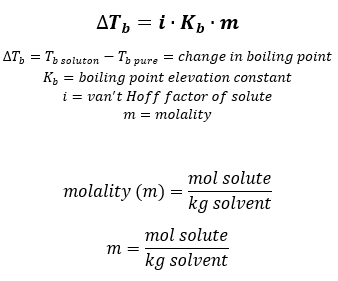# Problem: What is the boiling point of a solution of 9.04 g of I2 in 75.5 g of benzene, assuming the I2 is nonvolatile?(a) Outline the steps necessary to answer the question.(b) Answer the question

🤓 Based on our data, we think this question is relevant for Professor Rines' class at PBCC.

###### FREE Expert Solution

To solve this problem, recall the formula of boiling point elevation from colligative properties.(a)

Step 1: Determine the solute and solvent then determine van’t Hoff factor of the solute and Kb & Tb of the solvent###### Problem Details

What is the boiling point of a solution of 9.04 g of I2 in 75.5 g of benzene, assuming the I2 is nonvolatile?

(a) Outline the steps necessary to answer the question.